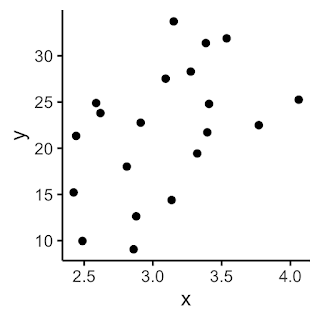# Colin Foster's Mathematics Education Blog

## 12 May 2022

### Learning times tables efficiently

Times tables can be a controversial subject. Can we help students to learn their tables in ways that promote conceptual understanding? This is my take on teaching times tables. I imagine there will be some strong opinions...

For many children, learning the times tables feels like a huge mountain to climb. And for those who have tried and feel that they have failed, going back and trying again fills them with dread. Perhaps all seems to go well in the beginning, with the 2s, 5s and 10s, say, but before long we reach the 6s, 7s and 8s, and it feels like every new fact that is mastered displaces an old fact that then becomes lost. As more and more facts are covered, the potential for muddling them up increases (e.g., $7 \times 8$: Is it $54$, or maybe $48$?), until the student really doesn’t have much idea which things they know and which they don’t. In the worst-case scenario, the only thing the child really trusts is skip-counting up from zero every time. And with skip-counting you only have to make one mistake for all of your remaining numbers to be wrong.

Teachers are highly strategic in the order in which they teach the tables: often 2s, 5s, 10s, 4s, … etc. But the effect of this is that the ‘hard stuff’ (6s, 7s, etc.) is delayed, so that when it arrives it can feel overwhelming and as though it is coming at learners far too quickly. I am not sure that learning one table after another like this – however carefully planned the sequence – is ideal (Note 1).

Here is a different way, that tries to build up from the multiplicative connections between the facts and deliberately avoids any addition/subtraction/skip-counting approaches, so as to build on the multiplicative structure of the tables and work more in harmony with that.

At first sight, there are 144 facts to learn:

But, of course, this is highly deceptive, and it is nowhere near as bad as this. Because of the commutativity of multiplication ($a \times b \equiv b \times a$, see Foster, 2022), we can immediately delete nearly half of these facts: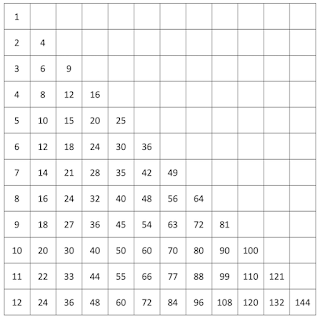Everyone knows their 1-times table (which is almost as easy as the 0-times table, Note 2), so we can grey those out. And I will assume that the 2s (the even numbers), 5s, 10s and 11s (at least up to $9 \times 11$) are also known, or easily learned, and so I’ve marked those in green below: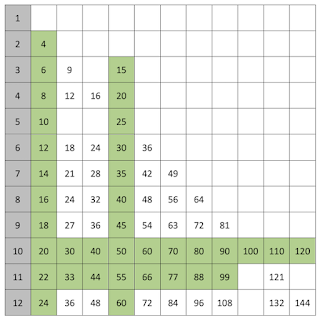So, from the original 144, this now leaves just 30 which need some teaching. And these are the tougher ones. Because of how the picture looks at this point, the best way to tackle this, I think, is not to go table by table (Note 3), but to exploit the structure a bit more strategically. In particular, we want to begin with the highest-leverage multiplication facts – the ones that help most with getting others. When students arrive, say, at secondary school and clearly ‘do not know their tables’, it is basically these 30 that are the problem. Convincing them that their difficulty is not a functionally infinite number of unknown facts but a relatively small number can be helpful. (It really is not like having to memorise a telephone directory!) And starting with the ones most likely to be of immediate help seems to make sense.

Big claim: The most useful of these remaining products to know are the eight squares in red below: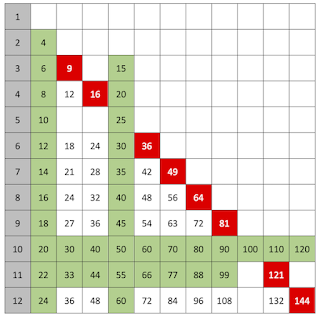In desperate circumstances, where students have repeatedly tried without success to master tables, I have been known to (reluctantly) settle for just knowing the squares. The beauty of the squares is that they march diagonally through the table, and so they really take you deep in amongst all the difficult facts. If you know the squares, the difficult products you don’t know are often only a step away.

For example, if you know that $8 \times 8 = 64$, then $7 \times 8$ must be $64-8 = 56$.
Or, if you know that $7 \times 7 = 49$, then $7 \times 8$ must be $49+7 = 56$.

So, the squares are really high-leverage facts to know, and I wouldn’t do anything else on the multiplication facts until the student knows these 8 squares. However, I am not really advocating pushing things like $7 \times 8 = 8 \times 8-8$, because students find this reasoning hard (Do I subtract 8 or 7?), and it breaks with the multiplicative theme.

So, instead, I would build differently from the squares:

$6 \times 3$ is half of $6 \times 6$ or double $3 \times 3$
$4 \times 8$ is half of $8 \times 8$ or double $4 \times 4$
$6 \times 12$ is half of $12 \times 12$ or double $6 \times 6$

This is really powerful. Mental doubling and halving may need some work, but that is very important anyway, so I am happy to be dependent on that (see Francome, 2020).

So, now we have three more facts, in orange below:Knowing that $6 \times 6 = 36$ is the single most powerful fact in the entire tables square, so long as you are able to mentally break down the 6s into 2s and 3s. Students who haven’t had much practice doing this ‘prime decomposition’ find it initially difficult, but this is at the heart of how multiplication works, so is an important awareness, and, with practice, it allows students to see why all the 36s in the table are equal (there are no 'coincidences' in the multiplication table):

$4 \times 9 = (2 \times 2) \times (3 \times 3) = (2 \times 3) \times (2 \times 3) = 6 \times 6 = 36$
So, $8 \times 9 = 2 \times 4 \times 9 = 2 \times 36 = 72$ and
$3 \times 12 = 6 \times 6$ (double the 3, halve the 12) $= 36$

These are in gold below: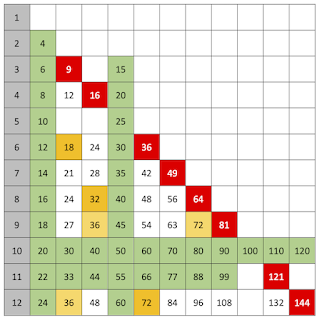Next, I would do 12, 24, 48 and 96. If you learn that $3 \times 4 = 12$ (which most students will know), then $3 \times 8$ (double the 4), $6 \times 4$ (double the 3), $12 \times 4$ (double the 3 twice), $6 \times 8$ (double the 3 and double the 4) and $12 \times 8$ (double three times) all come along without too much trouble if students are fluent doublers - and only the the last one of these involves any 'carrying' when doubling.

This means that when students are stuck on $6 \times 8$, the prompt would not be to count up in 6s or work from the nearest multiple of 6 or 8 they can think of (e.g., $6 \times 10$). It would be: Do you know $\textit 3 \times \textit 4$? (Both numbers are doubled, so the answer must be 12 double-doubled, which can be done easily mentally, without any 'carrying'.)

These six are in blue below, so, by this point, we have dealt with 20 of the tricky ones and there are just 10 left.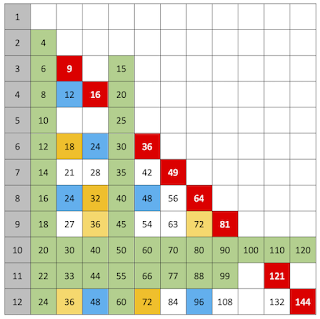The remaining ones are all ‘hard’, and we need to take time and care over these. I think I would spend 50% of my total energies on these 10.

There is 21, 42, 84 and 63 (in purple below), which all come from $3 \times 7$, which therefore needs to be learned. Then, given $3 \times 7$, we can do $6 \times 7$ (double), $12 \times 7$ (double twice) and $9 \times 7$ (triple). (None of these scalings is hard to do quickly mentally, as none involves any 'carrying'.)

Then there is 28 and 56 (in yellow below), where $8 \times 7$ is just double $4 \times 7$ (which is just double $2 \times 7$).

And then we have 27, 54 and 108 (in pink below), which come from $3 \times 9$, which needs to be learned (perhaps as $3^3$). We have $6 \times 9$ (double) and $12 \times 9$ (double twice).

Which just leaves 132 to remember (or know as $11^2 + 11$, which is possibly easier than double $6 \times 11$). I think this is probably the least connected of all of the multiplication facts, and so perhaps the hardest to remember.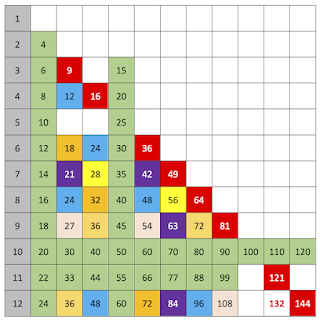So, in conclusion, this means that the only ones that potentially 'need' memorising are these 12:

$3^2, 4^2, 6^2, 7^2, 8^2, 9^2, 11^2, 12^2, 3 \times 4, 3 \times 7, 3 \times 9$ and $11 \times 12.$
And, if you have the 3-times table, then that reduces this list to just the other 7 squares and $11 \times 12$, which really feels manageable. It does show the power you get from knowing the squares (Note 4).

I think the key to supporting all of this is in the kind of prompts that you provide when a student is stuck. Rather than asking them to figure it out from ‘anything relevant that you know’, or waiting patiently while they skip-count up from zero, with this approach you have a clear plan for how they might be getting from known things to unknown. With practice, figuring out something like $7 \times 8$ by saying ‘double $7 \times 4$, which is 28, so that's 56’ can be extremely quick, and the more you do this the more you are incidentally practising doubling. (And this is one of the trickiest ones, because doubling 28 involves a mental 'carry'.) Of course, nothing will be as fast as ‘just knowing’, but, where that has repeatedly failed for a student, then this kind of approach may help. And I would teach it to everyone for the sake of understanding the multiplicative connections (Note 5). I certainly prefer to spend energy on this than on those one-off mnemonics, like ‘5-6-7-8’ for $56 = 7 \times 8$, which are flukes that don't generalise.

Here is my attempt at a (rather messy) summary of where everything comes from (pdf version):

In conclusion, I am not suggesting that any of this is easy, especially with students who have experienced repeated failure with tables or have developed ‘tables anxiety’. There is no quick, easy fix. And I’m not saying that I think this approach is definitely the best (e.g., I don’t make anything much of the 9s, which can be fairly easy to learn). But, I think that if you work through in this order you at least get the highest-leverage facts (e.g., the squares) before the lower-leverage ones (e.g., $11 \times 12$). However, if you have a better order - or entire approach - please put it in the comments below!

### Questions to reflect on

1. What are your best strategies for teaching the multiplication tables? Do you work differently with older learners who have previously been unsuccessful learning their multiplication tables?

2. What are the pros and cons of the different approaches you have tried?

3. What do you think of the scheme I have outlined above? Please respond in the comments if you can improve on it.

### Notes

1. For some great tables tasks that focus on conceptual understanding, see Faux (2018). See also the Position Statement on 'The Teaching and Learning of Multiplication Bonds' from the Joint ATM/MA Primary Group: https://www.m-a.org.uk/resources/1multiplicationbondsATMMA.pdf

2. Of course, except for the $1 \times n = 1 + n$ error, which seems to be particularly common with $1 \times 1 = 2$.

3. In the time of the Numeracy Strategies in the UK, everyone seemed to be chanting up and down in multiples on 'counting sticks', but I worry that that doesn't always help learners to remember which numbers belong in which tables. Once you move on to a new table, you trample all over numbers that have been learned in previous tables, with different but similar numbers appearing, and this interference makes it highly muddling for many students. It also feels 'additive' rather than 'multiplicative'.

4. Of course, some of the squares in this list could be derived from others in the list (e.g., $6^2$ is double-double $3^2$), but I tend to think that they are all important enough to know in their own right. But, if you disagree, then you could further reduce the list of base facts to just these nine: $3^2, 4^2, 7^2, 9^2, 11^2, 3 \times 4, 3 \times 7, 3 \times 9$ and $11 \times 12$, and get everything from just them.

5. The other advantage of 'just knowing' the tables, rather than working them out (even very quickly) is, of course, that you can work backwards, and when you see, say, 56, you immediately think 7s and 2s ($2^3 \times 7$). I think the kind of approach I've outlined here, focused on scaling up, rather than repeated addition, potentially helps with this, because, when you see 56, you are more likely to think 'double 28', and that can take you back to $14=2 \times 7$ and, via $4 \times 7$, to $8 \times 7$, so all the 'reverse doubling' helps to make visible the multiplicative structure that is there. Whereas thinking that 56 may be 7 or 8 more or less than some other half-remembered number doesn't do much for you.

### References

Faux, G. (2018). Tables together. Association of Teachers of Mathematics.  https://www.atm.org.uk/shop/act114pk/Tables-Together-e-book/DNL170

Foster, C. (2022). Getting multiplication the right way round. Mathematics in School, 51(2), 16–17. https://www.foster77.co.uk/Foster,%20Mathematics%20in%20School,%20Getting%20multiplication%20the%20right%20way%20round.pdf

Francome, T. (2020). Random chants: Generating a lot from a little using Excel. Mathematics Teaching, 274, 28-30.

1.Why 11 and 12? Don't we need to go only to 10? 11 and 12 come from the old days when there were 12 pennies in a shilling, etc, surely.

WR (it wouldn't let me set up a Name/URL: it said 'invalid URL', which is not true.)

1.I think there are some arguments for going up to 12, to do with 12 having lots of factors, so there's some structure you see beyond 10 which you wouldn't otherwise. There's also an argument for going past 10 just so that people don't see 10 as a hard stop (like going past 1 when working with fractions). And also for telling the time it's quite important to know 12 x 5 = 60. But I agree the need is less than it was.

(Sorry about the 'invalid URL' - I'll look into that!)

2.There are many interesting ideas here, none of which I would disagree with. This sounds like something of a double negative, however, I want to suggest other tasks, as positive additions to these you have suggested within an interconnected framework. I suggest multiplication and division need connecting just as multiples, divisors and factors need connecting. I thing x2, x4 and x8 need connecting, as does halves, quarters and eighths need connecting. I 100% agree squaring and square numbers are very important constructs and coming to recognise how square number have odd amounts of factors. How times tables and sequences are connected and how even numbers are connected to odd numbers.

3.(Please note that the comment above was from Mike Ollerton - for some reason the system labelled it 'Anonymous'.)

4.I spent three consecutive years working with the weakest mathematicians in Y5. All left the year group with rapid recall of multiplication facts. Here's how... First, we used a little deck of flashcards per pair of kids to learn the 2s and 5s rapidly. (Kids quiz their partner. Wrong answers go on a pile to be re-asked.) Once these were solid (and not before), we created a new set with 3s and 4s. Any flashcards that are consistently answered correctly by both partners get removed from the deck.) You slowly add sets of facts (but only new facts so, for example, by the time you get to the 7s - the last set - you only need to include 7x7).

The key things are (a) this needs to be done every day for five minutes, (b) encourage quick guessing and answer showing, and (c) put wrong answers on the re-ask pile, so kids are seeing these ones again.

Oh, and I don't bother with 11s and 12s. Beyond school accountability purposes, there is little purpose to them.

No wizardry needed. Just a set of flashcards per pair and lots of zero-stakes little-and-often practice. That's it.

### Lines of not-very-good fit

Does anyone teach lines of best fit 'properly' in lower secondary school? I think whenever I’ve seen this concept taught, or taught ...